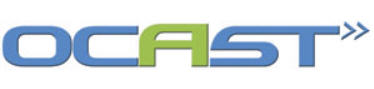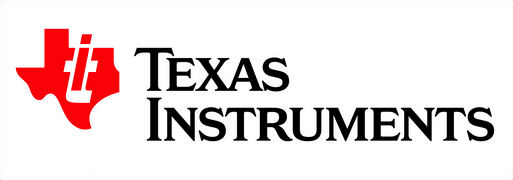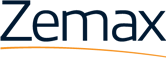## slider-spectroscopy2

The Diffraction Grating

After light passes through the slit, a set of lenses or mirrors collimates the light (makes all light rays parallel to the optical axis) and delivers the light to the diffraction grating. A diffraction grating consists of a material containing a periodic variation in one of its optical properties. The diffraction grating separates the wavelength components of the light by directing each wavelength into a unique output angle. The change in output angle as a function of wavelength, called the angular dispersion, plays an important role in determining the wavelength resolution of the spectrometer. The period of the variation in the optical property of the grating strongly determines the available angular dispersion. The efficiency of the diffraction grating determines the amount of optical power available to the DMD (in the Nano) or detector array as a function of wavelength. The geometry of the periodic variation strongly influences the available efficiency. Both the period and geometry of the grating must be carefully selected in order to meet the needs of your spectroscopic application.

Two different types of gratings can be used for spectroscopic applications. A ruled grating consists of a material into which a large number of parallel grooves are etched and then coated with a highly reflective material such as gold. A holographic grating is created by interference of two laser beams within a responsive material which results in a periodic variation in the refractive index of the material. Many processes can produce holographic gratings in a variety of materials, though the most common type found in spectrometers consists of a glass substrate exposed to interfering ultraviolet beams. Holographic grating can provide much higher angular dispersion due to the ability to write variations with very small periods, perform better in the ultraviolet end of the spectrum, and can be written onto curved surfaces to provide focusing capabilities in addition to the angular dispersion. However, holographic gratings are less efficient and more expensive than ruled gratings and absorption becomes an issue in the infrared end of the spectrum. Therefore, spectrometers operating in the infrared typically employ ruled gratings.

Both ruled and holographic gratings work on the same fundamental diffraction principles and are generally governed by the same sets of equations. Therefore, the discussions of angular dispersion, resolution, and efficiency apply to both types of gratings.

Angular Dispersion and Resolution

The fundamental operation of the diffraction grating is depicted in Figure G-1. The period of the grating is given by d, though most gratings are specified by the groove density D = 1/d quoted in typical units of grooves/mm. Incoming light strikes the grating at an incident angle α and leaves the grating at a diffracted angle β. Unlike a flat mirror, β does not equal α. In fact, the grating diffracts light into several different angles, called diffraction orders, each with a different diffraction efficiency. The condition given by α = β from Snell’s Law of reflection corresponds to the m = 0 diffraction order. The angles βm corresponding to the m = 1, 2, and higher order diffractions are found using the following fundamental grating equation:

sin(α) + sin(β) = mλD10-6     (1)

where the wavelength λ is in nanometers and the groove density D  is in grooves/mm. As the wavelength varies, so the does the output angle, and thus a range of wavelengths are deflected into a range of different angles and spatially separated, allowing the spectrometer to act on small ranges of wavelengths individually.

The resolution achieved by a spectrometer depends on the angular dispersion provided by the grating, defined as the change in β due to a change in λ, or dβ/dλ, and how the varying angles image onto the detector array or DMD. Taking the derivative of (1) gives

∂β/∂λ = (mD∙10-6) / cos(β)                           (2)

Note that a larger groove density D increase the angular dispersion, as does the use of higher diffraction orders. After diffraction by the grating, focusing optics direct the now dispersed light onto the linear detector array or DMD. The focusing optics may be the curved surface of the substrate of the grating or a combination of external lenses or mirrors. The optics convert the angular dispersion into a linear dispersion at the target surface, defined as the change in position on the surface as a function of the wavelength, as shown in Figure G-2. In general, the linear dispersion is given by

∂λ/∂x = (cos(β)∙10-6) / mDf                       (3)

where f is the effective exit focal length of the optics in mm and ∂x is the distance across the target surface in mm. Equation (3) applies exactly only to wavelengths incident perpendicular or very nearly perpendicular to the target surface. For other wavelengths, Equation (3) must be modified to account for the tilt angle γ between the wavelength’s ray and the surface, as shown in Figure G-2. The modified equation becomes

∂λ/∂x = cos(β) (cos(γ))2 ∙106 / (mDf)         (4)

where f is the focal length of the ray perpendicular to the surface.

Equation (4), along with information about the detector array or DMD, provides the information necessary to determine the wavelength range and the minimum possible wavelength resolution of the spectrometer.

To determine the wavelength range, the linear dispersion is multiplied by the length LS of the active surface, effectively shown in Fig G-3(a). For a linear detector array, LS is the number of detectors times the average width of the detectors. For a DMD, the number of mirrors in one row of the DMD times the horizontal dimension of the mirrors gives LS. Using Equation (3) for simplicity, the wavelength range of the spectrometer is given by

λmax - λmin = LS ∂λ/∂x= LS cos(β)∙10-6/mDf               (5)

A longer detecting surface or a smaller linear dispersion (spreading a range of wavelengths ∂λ over a smaller distance ∂x) allows the spectrometer to analyze more wavelengths in a single measurement.

The wavelength resolution of the spectrometer has a theoretical minimum and a practical minimum value. The resolving power R of the grating itself defines the theoretical minimum resolution. The resolving power is defined as

R = λctr / Δλ

where Δλ is the minimum separation between two spectral lines that can be observed as distinct, individual lines at the output of the grating when operated around a central wavelength λctr. In diffraction theory, the output of the grating at a given wavelength produces a sinc2 function in space, which consists of an intense central lobe of some width and many less intense side lobes. The Rayleigh criterion dictates that two sinc2–shaped patterns can be distinguished from each other once the peak of the main lobe of one pattern moves to the zero in between the main lobe and the first side lobe of the second pattern. For spectrometers, this criterion and the geometry of the spectrometer sets the resolving power as

R = Wg ( sin(α)+sin(β)) / (10-6. λ)               (6)

where Wg is the illuminated width of the diffraction grating in mm. In practice, the minimum resolution obtained for a given spectrum depends more on the parameters of the detector array or DMD than the resolving power, as well as the width of the entrance slit.

An estimate of the practical resolution of the spectrometer combines the linear dispersion with the physical dimensions of the detector array or the DMD, effectively shown in Figure G-3(b). In either case, a group of wavelengths incident on a single detector or on a single DMD mirror are measured simultaneously as a single unit with total power attributed to the nominal central wavelength of the group. Therefore, an estimate of the wavelength resolution of the spectrometer, considering only the grating and the analyzing array parameters, can be approximated by

∆λ ≈ Lpixel ∂λ / ∂x                   (7)

where Lpixel is the dimension of the detector or mirror along the horizontal direction, which in most cases is very close to the distance between detectors or mirrors. A better resolution (smaller Δλ) is obtained by either decreasing the pixel dimensions (smaller detectors or mirrors) or by increasing the linear dispersion of the grating – distributing the same number of wavelengths ∂λ over a much larger distance ∂x. In some cases, careful measurement can provide a sub-pixel/mirror resolution smaller than that in Equation (7), though in general the limit set in Equation (7) holds true. Combining the results of Equations (7) and (5) illuminates an important trade-off in the design and operation of spectrometers. To get better resolution requires a higher linear dispersion, while improving the range of the spectrometer requires a smaller linear dispersion. To balance the two conditions requires care in selecting the dimensions of the analyzing array for a given choice of groove density for the grating.

Power Efficiency

Since the accuracy and speed of a spectroscopic measurement depend on the amount of optical power available to the detector, the grating directs must be able to efficiently direct optical power into the chosen diffraction order sent to the detector array or DMD. The power delivered to a given diffraction order depends on the geometry of the periodic variation of the optical properties of the grating. For gratings with symmetrical geometries – a symmetric triangle pattern for ruled gratings, or a sinusoidal pattern for holographic gratings – the m = 0 order contains, in general (though not for all grating cases), a larger percentage of the optical power, and the m = 1 and higher orders used by the spectrometer contain only limited power. Changing the geometry of the variation, called “blazing,” can optimize the power coupled into the diffraction order of choice. The geometry of a blazed ruled grating is shown in Figure G-4, where θ is defined as the blaze angle. Since the output angle β depends on the incident wavelength, the blaze angle optimizes the diffraction efficiency only in a range of wavelengths around the blaze wavelength λB. Figure G-5 shows the center of the diffraction enveloped can be moved to coincide with one of the diffraction orders. As a general rule, the efficiency at λB can be greater than 85%, and the diffraction efficiency falls to 50% of the maximum efficiency at 0.6λB and 1.8λB. The decrease in efficiency imposes an additional constraint on the overall operating range of the spectrometer. It is important to select the correct blaze wavelength for a given application. Based on the typical 50% efficiency range, the selected blaze wavelength should lie within the lower half of the desired operating wavelength range of the application.

## clients logos table

 Optecks’ Research funded in part by:Optecks is an Authorized Design House at:Find us on zemax.com